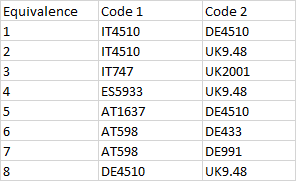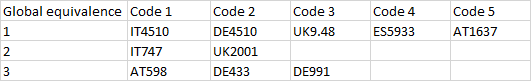# Joining different pairs of value equivalences into "global equivalences"

Hello,

I’m trying to do a certain transformation and I’m not sure how to tackle it. The origin table is a list of pairs of equivalences, which take place between two codes:In equivalence “1”, code 1 “IT4510” is equal to code 2 “DE4510”, in equivalence “2” code “IT4510” is equal to code 2 “UK9.48” and so on.

If “IT4510” is equal to both “DE4510” and “UK9.48”, this means that “DE4510” and “UK9.48” are also equal to each other. If we look at equivalence “4”, we can see that code 1 “ES5933” would also join this group, as it is equivalent to “UK9.48”.

Equivalence “3” however contains two codes that do not appear in any of the other lines, so in this case there’s no more cross-equivalences to make.

The desired output table shows the total “global equivalences” that really occur in all these pairs of equivalences. It would look like this:In total, there are 3 “global equivalences”, because all the cross-equivalences seen in the origin table are taken into account and linked together, and each unique code only appears once now. Note that both codes of equivalence “8” of the origin table are already included in global equivalence “1”.

I think the solution involves iterating each row, but the iterated row needs to have other multiple rows into account in order to obtain the global equivalence, and that’s where I’m stuck.

Thanks very much,

Roberto

Hi Roberto,

do you know if the max number of codes in one equivalence group can never be greater than a particular number, e.g. 10, or not?

Also, a small sample of test data would be helpful (instead of the image in your post).

Hello Dmitry,

The maximum number of equivalences in one group is equal to the number of different prefixes that codes can have (for this example it’s 5 - IT, ES, AT, DE, UK), as not two codes sharing the same prefix can be inside an equivalence group (there was an incongruence in the image of the table I posted before, it’s solved in the excel spreadsheet I attach). In the real case I’m treating at the moment, the number o different prefixes at the moment is 6, but that number could change.

I attach an excel spreadsheet with three sheets:

• “Input”: with the equivalence pairs
• “Output”: with global equivalences
• “Output - same prefix per column”: the codes are organized in columns depending on the prefix they have, I forgot to mention this transformation in the opening post).

Equivalence groups.xlsx (9.8 KB)

Some additional info on how the input data comes (“Input” sheet in the Excel): the different pairs of equivalences always come in the same order - in Column “Code 1” the different codes with prefixes (if present) will always appear in the following order: IT, ES, AT, DE, UK. And an equivalence pair will only appear once - there can’t be another equivalence which is the same as one before but with “Code 1” and “Code 2” swapped, this can’t happen.

If there’s anything else that I need to explain further, please let me know.

Thanks a lot!

Roberto

I would just assume that a reasonable fixed number of recursive iterations should always be sufficient. If that number is rather high (e.g. >10), then a recursive loop can be arranged as described here: How to do recursive loops in EasyMorph.

Each iteration looks up an equivalence group with a lower group number, if it exists. When an equivalence group with a lower group number is found, the original group number is replaced with the lower one. This logic merges equivalence groups into bigger groups.

Note that module “Group” creates an output dataset with exactly the same column names as its input dataset. That allows simply chaining calls to this module one after another.

See the example below.

pairwise-grouping.morph (11.3 KB)

Module “Main”

Module “Group”

2 Likes

Thanks very much Dmitry, this is perfect!Regards,

Roberto